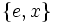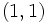# Subgroup structure of M16

View subgroup structure of particular groups | View other specific information about M16

To describe subgroups, we use the defining presentation given at the beginning:$M_{16} = \langle a,x \mid a^8 = x^2 = e, xax = a^5 \rangle$.

## Tables for quick information

FACTS TO CHECK AGAINST FOR SUBGROUP STRUCTURE: (group of prime power order)
Lagrange's theorem (order of subgroup times index of subgroup equals order of whole group, so all subgroups have prime power orders)|order of quotient group divides order of group (and equals index of corresponding normal subgroup, so all quotients have prime power orders)
prime power order implies not centerless | prime power order implies nilpotent | prime power order implies center is normality-large
size of conjugacy class of subgroups divides index of center
congruence condition on number of subgroups of given prime power order: The total number of subgroups of any fixed prime power order is congruent to 1 mod the prime.

### Table classifying subgroups up to automorphism

Automorphism class of subgroups List of subgroups Isomorphism class Order of subgroups Index of subgroups Number of conjugacy classes(=1 iff automorph-conjugate subgroup) Size of each conjugacy class(=1 iff normal subgroup) Total number of subgroups(=1 iff characteristic subgroup) Isomorphism class of quotient (if exists) Subnormal depth Nilpotency class
trivial subgroup$\{ e \}$ trivial group 1 16 1 1 1 M16 1 0
derived subgroup of M16$\{ e, a^4 \}$ cyclic group:Z2 2 8 1 1 1 direct product of Z4 and Z2 1 1
other subgroups of order two$\{ e, x \}$,$\{ e, a^4x \}$ cyclic group:Z2 2 8 1 2 2 -- 2 1
center of M16$\{ e, a^2, a^4, a^6 \}$ cyclic group:Z4 4 4 1 1 1 Klein four-group 1 1
other cyclic subgroup of order 4$\{ e, a^2x, a^4, a^6x \}$ cyclic group:Z4 4 4 1 1 1 cyclic group:Z4 1 1
V4 in M16$\{ e, x, a^4, a^4x \}$ Klein four-group 4 4 1 1 1 cyclic group:Z4 1 1
Z8 in M16$\{ e,a,a^2,a^3,a^4,a^5,a^6,a^7 \}$$\{ e,ax,a^6,a^7x,a^4,a^5x,a^2,a^3x\}$
cyclic group:Z8 8 2 2 1 2 cyclic group:Z2 1 1
direct product of Z4 and Z2 in M16$\{ e,a^2,a^4,a^6,x,a^2x,a^4x,a^6x \}$ direct product of Z4 and Z2 8 2 1 1 1 cyclic group:Z2 1 1
whole group all elements M16 16 1 1 1 1 trivial group 1 1
Total (9 rows) -- -- -- -- 10 -- 11 -- -- --

### Table classifying isomorphism types of subgroups

Group name GAP ID Occurrences as subgroup Conjugacy classes of occurrence as subgroup Automorphism classes of occurrence as subgroup Occurrences as normal subgroup Occurrences as characteristic subgroup
Trivial group$(1,1)$ 1 1 1 1 1
Cyclic group:Z2$(2,1)$ 3 2 2 1 1
Cyclic group:Z4$(4,1)$ 2 2 2 2 2
Klein four-group$(4,2)$ 1 1 1 1 1
Cyclic group:Z8$(8,1)$ 2 2 1 2 0
Direct product of Z4 and Z2$(8,2)$ 1 1 1 1 1
M16$(16,6)$ 1 1 1 1 1
Total -- 11 10 9 9 7

### Table listing number of subgroups by order

Group order Occurrences as subgroup Conjugacy classes of occurrence as subgroup Automorphism classes of occurrences as subgroup Occurrences as normal subgroup Occurrences as characteristic subgroup
1 1 1 1 1 1
2 3 2 2 1 1
4 3 3 3 3 3
8 3 3 2 3 1
16 1 1 1 1 1
Total 11 10 9 9 7